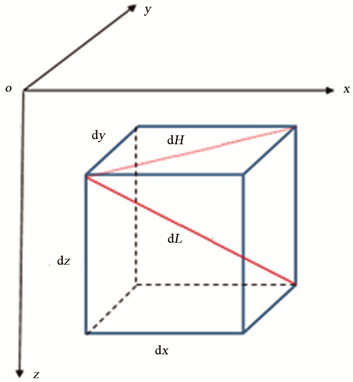# 连续油管下入作业力学特性研究Study on Mechanical Properties of Coiled Tubing Setting Operation

DOI: 10.12677/JOGT.2019.415068, PDF, HTML, XML, 下载: 274  浏览: 372

Abstract: During the operation of coiled tubings, great influence would be induced because of the internal and external pressure of downhole fluid, tubing instability and bending as well as other factors. Based on the well depth coordinate parameters of drilling rig, the geometric model of well trajectory was established, and the numerical integration method of Simpson quadrature formula was used to calculate the well shaft coordinate parameters. Based on the obtained coordinate parameters, the mechanical properties of coiled tubing, such as annulus pressure, axial force and bending moment, were analyzed and calculated respectively. Based on the actual borehole trajectory parameters, the mechanical properties were analyzed with each equation. It provides a basis for analyzing the mechanical properties of coiled tubings.

1. 前言

2. 连续油管下入分析模型建立Figure 1. The micro-elemental model of well trajectory

$\left\{\begin{array}{l}\text{d}x=\mathrm{sin}\alpha \mathrm{sin}\phi \text{d}L\\ \text{d}y=\mathrm{sin}\alpha \mathrm{cos}\phi \text{d}L\\ \text{d}z=\mathrm{cos}\alpha \text{d}L\\ \text{d}H=\mathrm{sin}\alpha \text{d}L\end{array}$ (1)

$\left\{\begin{array}{l}x={\int }_{0}^{L}\mathrm{sin}\alpha \mathrm{sin}\phi \text{d}L\\ y={\int }_{0}^{L}\mathrm{sin}\alpha \mathrm{cos}\phi \text{d}L\\ z={\int }_{0}^{L}\mathrm{cos}\alpha \text{d}L\\ H={\int }_{0}^{L}\mathrm{sin}\alpha \text{d}L\end{array}$ (2)

3. 连续油管力学性能研究

3.1. 连续油管内外流体压力计算

${p}_{1,i+1}={p}_{1,i}+{\rho }_{1,i}g\left(\mathrm{cos}{\alpha }_{i}-\frac{{\lambda }_{1,i}{\upsilon }_{1,i}^{2}}{4g{r}_{1,i}}\right)\Delta {l}_{i}$ (3)

${p}_{2,i+1}={p}_{2,i}+{\rho }_{2,i}g\left(\mathrm{cos}{\alpha }_{i}-\frac{{\lambda }_{2,i}{\upsilon }_{2,i}^{2}}{4g{r}_{2,i}}\right)\Delta {l}_{i}$ (4)

3.2. 连续油管轴向力计算

${F}_{\text{e}\tau ,i-1}={F}_{\text{e}\tau ,i}+\left[{q}_{\text{e},i}\mathrm{cos}{\alpha }_{i}-\left({f}_{i}{N}_{i}+{f}_{1,i}+{f}_{2,i}\right)\right]\Delta {l}_{i}$ (5)

${F}_{\tau ,i}={F}_{\text{e}\tau ,i}-{p}_{1,i}{A}_{1,i}+{p}_{2,i}{A}_{2,i}$ (6)

3.3. 连续油管弯矩计算

$M=\left\{\begin{array}{l}EI{k}_{0,i}\text{\hspace{0.17em}}\text{\hspace{0.17em}}\text{\hspace{0.17em}}\text{\hspace{0.17em}}\text{\hspace{0.17em}}\text{\hspace{0.17em}}\text{\hspace{0.17em}}\text{\hspace{0.17em}}\text{\hspace{0.17em}}\text{\hspace{0.17em}}\text{\hspace{0.17em}}\text{\hspace{0.17em}}\text{\hspace{0.17em}}\text{\hspace{0.17em}}\text{\hspace{0.17em}}\text{\hspace{0.17em}}\text{\hspace{0.17em}}\text{\hspace{0.17em}}\text{\hspace{0.17em}}\text{\hspace{0.17em}}\text{\hspace{0.17em}}\text{\hspace{0.17em}}\text{\hspace{0.17em}}\text{\hspace{0.17em}}\text{\hspace{0.17em}}\text{\hspace{0.17em}}\text{\hspace{0.17em}}\text{\hspace{0.17em}}\text{\hspace{0.17em}}\text{\hspace{0.17em}}\text{\hspace{0.17em}}\text{\hspace{0.17em}}\text{\hspace{0.17em}}\text{\hspace{0.17em}}\text{\hspace{0.17em}}\text{\hspace{0.17em}}\text{\hspace{0.17em}}\text{\hspace{0.17em}}\text{\hspace{0.17em}}\text{\hspace{0.17em}}\text{\hspace{0.17em}}\text{\hspace{0.17em}}\text{\hspace{0.17em}}\text{\hspace{0.17em}}\text{\hspace{0.17em}}\text{\hspace{0.17em}}\text{\hspace{0.17em}}\text{\hspace{0.17em}}\text{\hspace{0.17em}}\text{\hspace{0.17em}}\text{\hspace{0.17em}}\text{\hspace{0.17em}}\text{\hspace{0.17em}}\text{\hspace{0.17em}}\text{\hspace{0.17em}}\text{\hspace{0.17em}}\text{\hspace{0.17em}}\text{\hspace{0.17em}}\text{\hspace{0.17em}}\text{\hspace{0.17em}}\text{\hspace{0.17em}}\text{\hspace{0.17em}}\text{\hspace{0.17em}}b\le 1\\ \sqrt{\frac{2EI{r}_{i}{d}_{n,i}}{3}\left(b-1\right)+\frac{6EI{r}_{i}{d}_{n,i}}{9}{\left(b-1\right)}^{2}+{\left(EI{k}_{0,i}\right)}^{2}}\text{\hspace{0.17em}}\text{\hspace{0.17em}}\text{\hspace{0.17em}}\text{\hspace{0.17em}}\text{\hspace{0.17em}}11.518\end{array}$ (7)

4. 结语

1) 根据钻机作业下的井深坐标参数，建立井眼轨迹几何模型，对井斜角、方位角函数简单的情况直接积分计算；当函数较为复杂时，可以采用复化辛普森求积公式的数值积分方法计算井眼轴坐标参数。

2) 对于已知井眼轴坐标参数的情况下，由环空压力、轴向力、弯矩等力学特性计算公式，可以求得任意井对应节点处的力学特性，为连续油管作业提供力学分析基础。

  王鹏, 王凤山, 张倩. 连续油管压裂管柱轴向载荷传递规律研究[J]. 石油矿场机械, 2016, 45(1): 20-23.  陈刚, 杨雪, 潘保芝, 等. 井眼轨迹计算及可视化研究现状[J]. 世界地质, 2015, 34(3): 830-841.  魏微. 复杂结构井井眼轨迹三维可视化技术研究[D]: [硕士学位论文]. 西安: 西安石油大学, 2012.  赵明炎. 水平井油套合压管柱力学分析[D]: [硕士学位论文]. 大庆: 东北石油大学, 2017.  程文. 连续油管力学特性分析与疲劳寿命研究[D]: [硕士学位论文]. 成都: 西南石油大学, 2016.  肖晖. 连续油管力学行为及低周疲劳寿命研究[D]: [硕士学位论文]. 成都: 西南石油大学, 2017.  张洪伟. 连续油管力学分析[D]: [硕士学位论文]. 青岛: 中国石油大学, 2010.